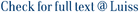We consider a discrete model in which particles are characterized by two quantities X and Y ; both quantities evolve in time according to stochastic dynamics and the equation that governs the evolution of Y is also influenced by mean-field interaction between the particles. We allow for discontinuous coefficients and random initial condition and, under suitable assumptions, we prove that in the limit as the number of particles grows to infinity the dynamics of the system is described by the solution of a Fokker–Planck partial differential equation. We provide the existence and uniqueness of a solution to the latter and show that such solution arises as the limit in probability of the empirical measures of the system.

Spatial dynamics in interacting systems with discontinuous coefficients and their continuum limits / Zanco, Giovanni Alessandro. - In: STOCHASTICS AND DYNAMICS. - ISSN 0219-4937. - 20:6(2020), pp. ---. [10.1142/S0219493720400080]

### Spatial dynamics in interacting systems with discontinuous coefficients and their continuum limits

#### Abstract

We consider a discrete model in which particles are characterized by two quantities X and Y ; both quantities evolve in time according to stochastic dynamics and the equation that governs the evolution of Y is also influenced by mean-field interaction between the particles. We allow for discontinuous coefficients and random initial condition and, under suitable assumptions, we prove that in the limit as the number of particles grows to infinity the dynamics of the system is described by the solution of a Fokker–Planck partial differential equation. We provide the existence and uniqueness of a solution to the latter and show that such solution arises as the limit in probability of the empirical measures of the system.
##### Scheda breve Scheda completa Scheda completa (DC)Mean-field interaction; Fokker–Planck equation; SDEs with discontinuous coefficients.
Spatial dynamics in interacting systems with discontinuous coefficients and their continuum limits / Zanco, Giovanni Alessandro. - In: STOCHASTICS AND DYNAMICS. - ISSN 0219-4937. - 20:6(2020), pp. ---. [10.1142/S0219493720400080]
File in questo prodotto:
File
SD-D-19-00153R1.pdf

Embargo fino al 06/06/2020

Descrizione: Aricolo - Versione post referaggio, non impaginata
Tipologia: Documento in Post-print
Licenza: DRM non definito
Dimensione 275.57 kB
Utilizza questo identificativo per citare o creare un link a questo documento: `http://hdl.handle.net/11385/199453`
•0
•0